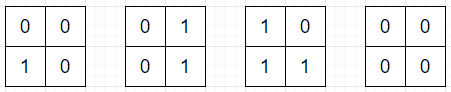# Program to count number of operations to convert binary matrix to zero matrix in C++

Suppose we have a binary matrix. Now consider an operation where we take one cell and flip it and all its neighboring cells (up, down, left, right). We have to find the minimum number of operations required such that matrix contains only 0s. If there is no solution, then return -1.

So, if the input is like

 0 0 1 0

then the output will be 3.To solve this, we will follow these steps −

• Define an array dir of size: 4 x 2 := {{1, 0}, {0, 1}, { - 1, 0}, {0, - 1}}
• const int inf = 10^6
• Define a function getPos(), this will take i, j,
• return i * c + j
• Define one function getCoord() this will take x
• Define one pair ret
• ret := x / c
• ret := x mod c
• return ret
• From the main method do the following:
• r := row count of matrix
• c := column count of matrix
• last := r * c
• for initialize i := 0, when i < r, update (increase i by 1), do:
• for initialize j := 0, when j < c, update (increase j by 1), do:
• Define an array dist of size 512 and fill with -1
• Define one queue q
• while (q is not empty), do:
• mask := first element of q
• delete element from q
• for initialize i := 0, when i < last, update (increase i by 1), do:
• Define one pair coord
• x := coord
• y := coord
• for initialize k := 0, when k < 4, update (increase k by 1), do:
• nx := x + dir[k, 0]
• ny := y + dir[k, 1]
• if nx and ny are not in range of matrix, then:
• pos := getPos(nx, ny)
• return dist

Let us see the following implementation to get better understanding −

## Example

Live Demo

#include
using namespace std;
int dir = {{1, 0}, {0, 1}, {-1, 0}, {0, -1}};
int c;
int r;
int last;
const int inf = 1e6;

int getPos(int i, int j){
return i * c + j;
}
pair getCoord(int x){
pair ret;
ret.first = x / c;
ret.second = x % c;
return ret;
}

int solve(vector>& matrix) {
r = matrix.size();
c = r ? matrix.size() : 0;
last = r * c;
for(int i = 0; i < r; i++){
for(int j = 0; j < c; j++){
mask ^= (matrix[i][j] << getPos(i, j));
}
}
vector dist(1 << 9, -1);
queue q;
while(!q.empty()){
q.pop();

for(int i = 0; i < last; i++){
pair coord = getCoord(i);
int x = coord.first;
int y = coord.second;

for(int k = 0; k < 4; k++){
int nx = x + dir[k];
int ny = y + dir[k];
if(nx < 0 || nx >= r || ny < 0 || ny >= c)
continue;
int pos = getPos(nx, ny);
}

}
}
}
return dist;
}
int main(){
vector> v = {{0, 0},{1, 0}};
cout << solve(v);
}

## Input

{{0, 0},{1, 0}}

## Output

3